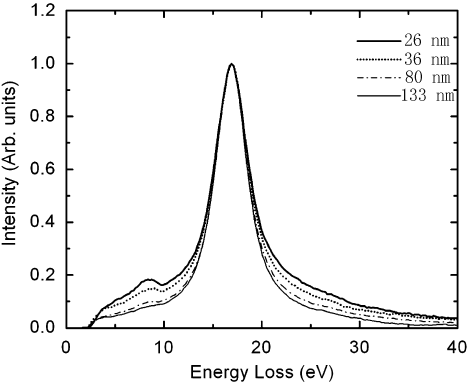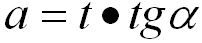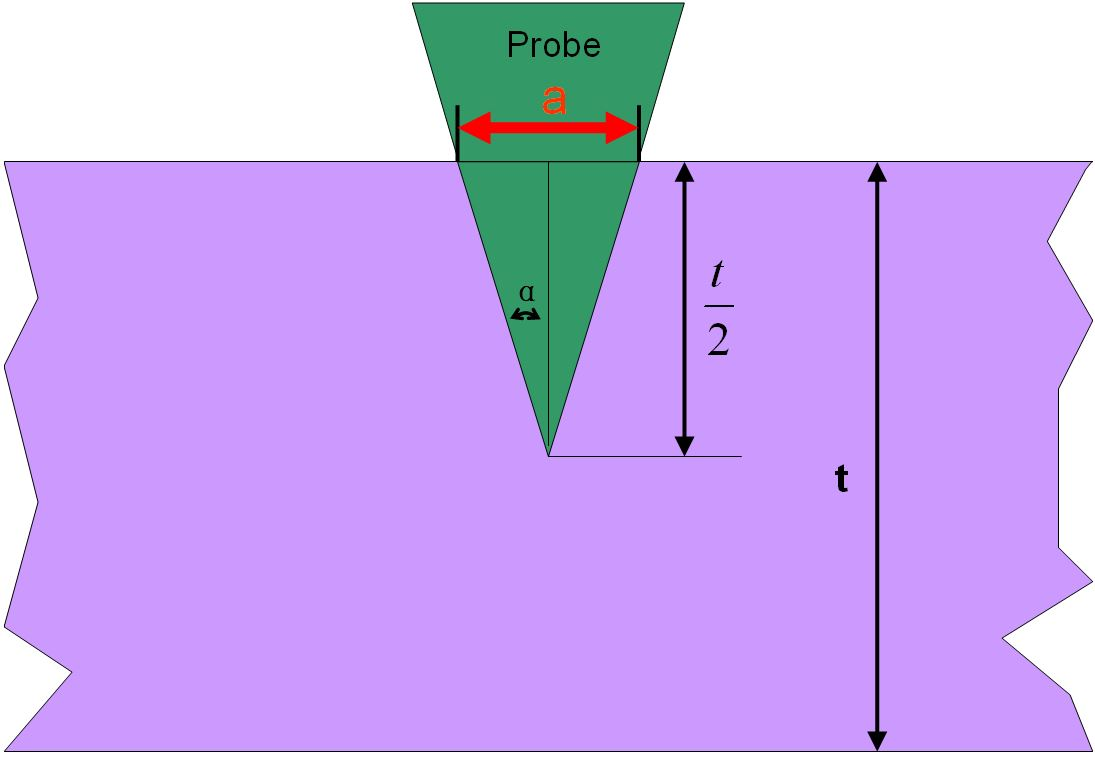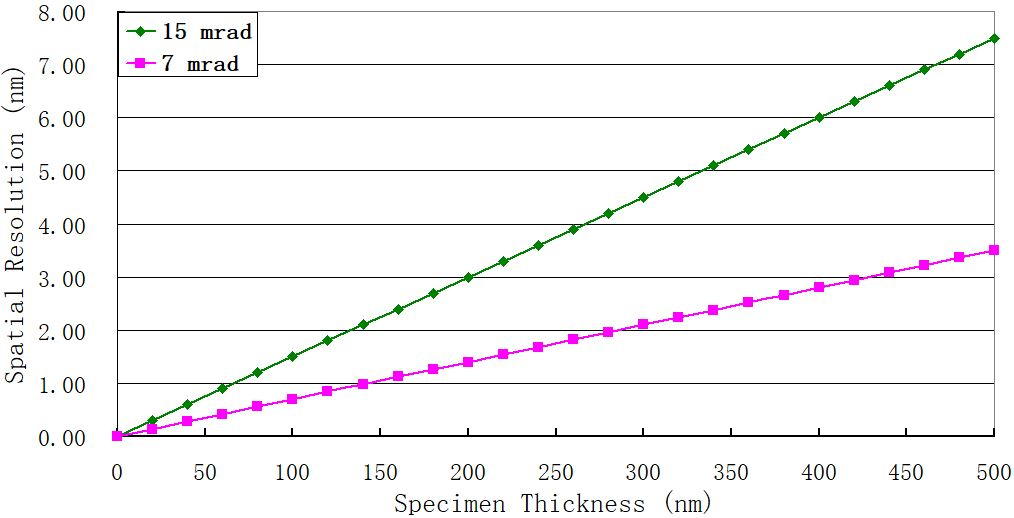Dependence of EELS Signal on TEM Specimen Thickness
- Practical Electron Microscopy and Database -
- An Online Book -Microanalysis | EM Book                                                                   https://www.globalsino.com/EM/

Mkhoyan et al.  presented SSDs (single scattering distributions) obtained from experimental EELS data from silicon (Si) specimens with four different thicknesses as shown in Figure 2401a. The band gap or electronic states in the gap presented in the figure were inaccurate due to the low energy-resolution. In their measurements, the energy resolution was 0.7 eV, and the channel size 0.22 eV so that it was impossible to quantify features located below 2 eV.Figure 2401a. SSDs obtained from experimental EELS data from Si specimens with four different thicknesses. Adapted from 

Note that the surface plasmon-loss (Is) has dependence on TEM-specimen thickness only when the specimen is extremely thin (e.g. <10 nm in general). On the other hand, for very thin TEM specimens, e.g. ≤30 nm for Si (≤0.25 λP), several surface-induced effects contribute to plasmon signal:
i) Radiative surface plasmons  locate just above bulk plasmon energies (EP).
ii) Coupling of surface plasmons  excited at the two surfaces.
iii) Coupling of surface and bulk plasmon excitation .
iv) Errors in calculations in the energy region of EP to 2EP due to angular effects. These errors are not considered in the Fourier-log deconvolution process that is the method normally used in the calculations.

In general, the requirements of TEM specimen thickness for EELS and EFTEM measurements are:
i) The specimens should be sufficiently thin to prevent any multiple inelastic scattering, but the degree of single inelastic scattering should be relatively high. For most materials, the optimized specimen thickness is in the range of 25-100 nm, depending on the average atomic number and the beam energy.
ii) To avoid surface effects, the specimen thickness cannot be less than 25 nm if low-loss EELS spectra are measured.

Figure 2401b shows that the background increases dramatically with increase of the thickness of TEM specimen, while the signal of Pt M4,5 edge decreases significantly. The highest signal in the range of these TEM specimen thicknesses for Pt occurs at 20 nm.Figure 2401b. EELS of Pt M4,5 edge.

Furthermore, the "local" EELS intensity also depends on the spatial resolution of STEM. However, the spatial resolution of the measurements in STEM mode can be estimated by the diameter of the probe irradiation on the specimen. Assuming the thickness of the specimen is t, then the spatial resolution is given by (see Figure 2401c):-------------------------------- 
where,
α -- The convergence semiangle.Figure 2401c. Diameter of the probe irradiation with specimen thickness t.

For instance, Figure 2401d shows the spatial resolutions at convergence semiangles 7 and 15 mrad, respectively, based on Equation 2401. Note that such convergence semiangles are used in many TEM systems.Figure 2401d. Spatial resolutions at convergence semiangles 7 and 15 mrad, respectively, based on Equation 2401.

 K.A. Mkhoyan, T. Babinec, S.E. Maccagnano, E.J. Kirkland, and J. Silcox, Separation of bulk and surface-losses in low-loss EELS
measurements in STEM, Ultramicroscopy 107 (2007) 345–355.
 R. Vincent, J. Silcox, Phys. Rev. Lett. 31 (1973) 1487.
 R.B. Pettit, J. Silcox, R. Vincent, Phys. Rev. B 11 (1975) 3116.
 C.H. Chen, J. Silcox, R. Vincent, Phys. Rev. B 12 (1975) 64.

 ;